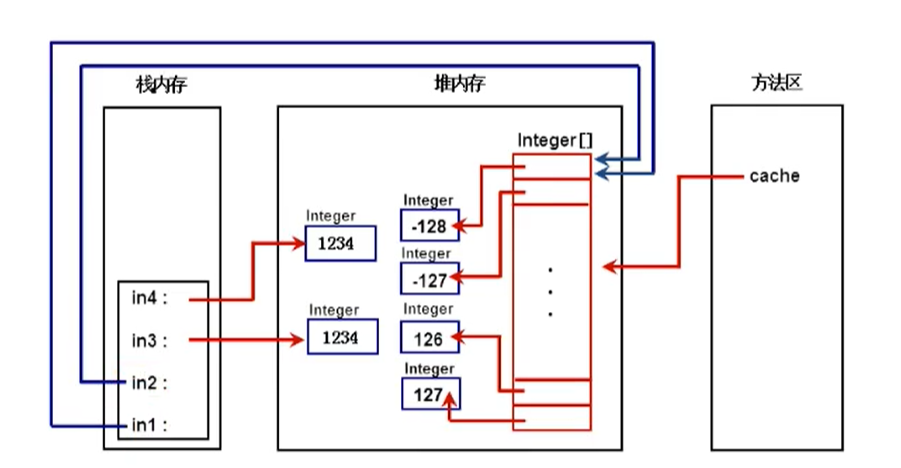# Java常用类

## 1.包装类

### 1.1什么是包装类？

Java 是面向对象的语言，但不是“纯面向对象”的，比如我们经常用到的基本数据类型就不是对象。

8种基本数据类型，分别对应一个包装类。包装类均位于java.lang包：

byte Byte
boolean Boolean
short Short
char Character
int Integer
long Long
float Float
double Double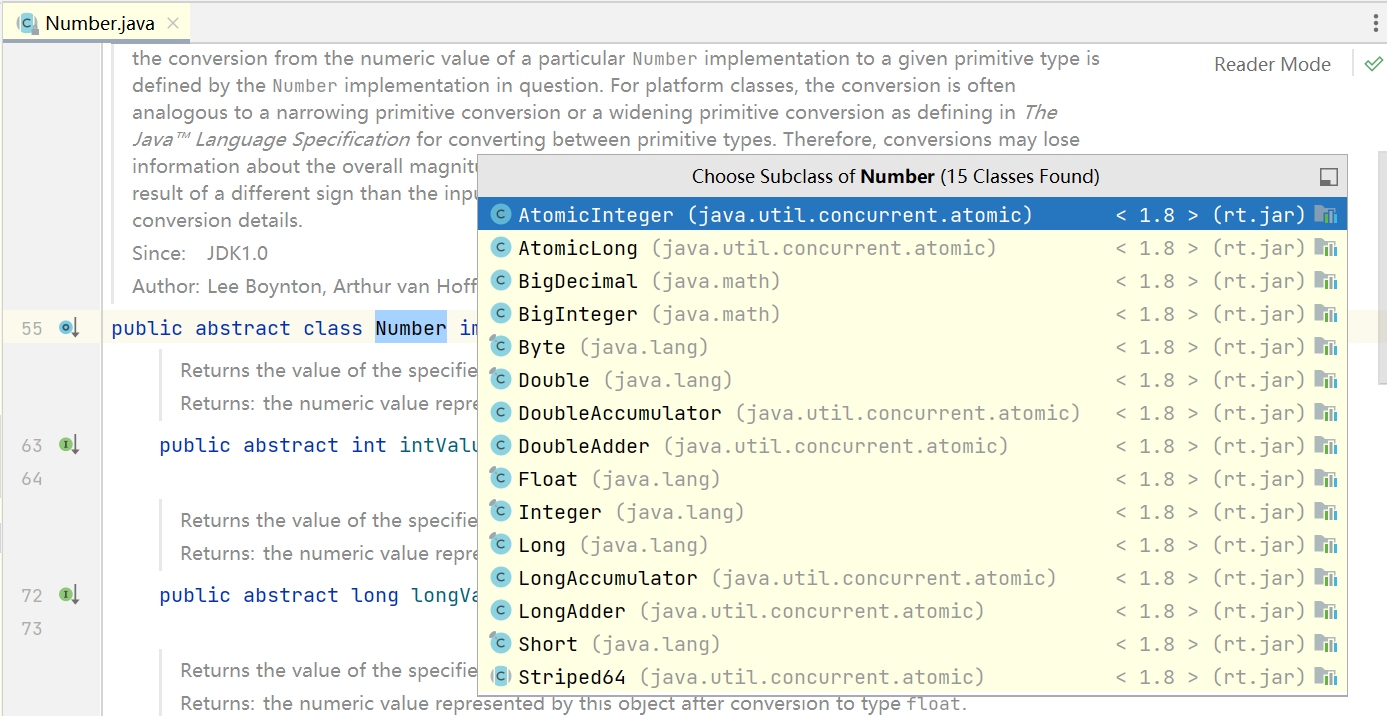### 1.2为什么要用包装类？（优点）

1. 某些方法的参数必须是对象，为了能让基本数据类型的数据能作为参数，因此提供包装类
2. 包装类还可以提供更多的功能
3. 其他特别重要的功能：比如可以实现字符串和基本数据类型之间的转换

``````package li.normalclass.warpper;

import java.util.ArrayList;
import java.util.Date;
import java.util.List;

public class TsetWarpper1 {
public static void main(String[] args) {
// 1. 某些方法的参数必须是对象，为了能让基本数据类型的数据能作为参数，因此提供包装类
List list = new ArrayList();

//自动装箱和拆箱从Java 1.5开始引入，目的是将原始类型值转自动地转换成对应的对象

System.out.println(list);
}
}
``````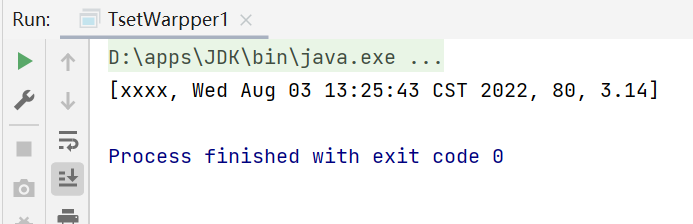``````package li.normalclass.warpper;

import java.util.ArrayList;
import java.util.Date;
import java.util.List;

public class TsetWarpper1 {
public static void main(String[] args) {
// 2. 包装类还可以提供更多的功能
System.out.println(Integer.MAX_VALUE);//2147483647

System.out.println(Integer.MIN_VALUE);//-2147483648

System.out.println(Integer.SIZE);//32bit

System.out.println(Integer.TYPE);//底层包装的基本数据类型
}
}

``````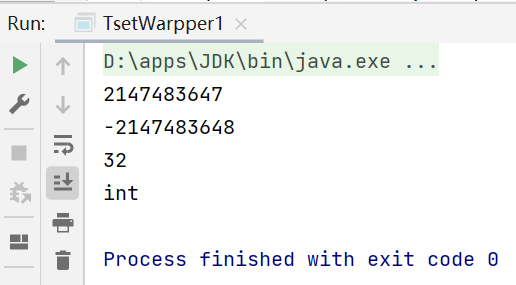``````package li.normalclass.warpper;

public class TsetWarpper1 {
public static void main(String[] args) {

//3. 其他特别重要的功能：比如可以实现字符串和基本数据类型之间的转换
//比如用户在网页表单输入的所有内容，java接收完之后会全部识别为String字符串类型，这时候就要我们将字符串根据需要转变为不同的数据类型
//注意字符串不能强制转换成数值类型，不能用强制转换

int age  = Integer.parseInt("18");
double pi = Double.parseDouble("3.14");
System.out.println(age);
System.out.println(pi);
}
}
``````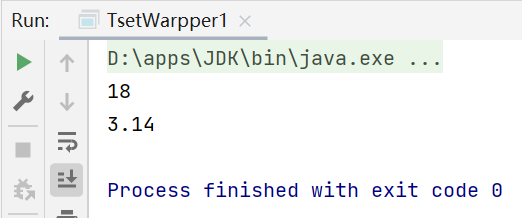### 1.3如何使用包装类

#### 1.3.1自动装箱和自动拆箱

• 自动装箱和自动拆箱就是将基本数据类型和包装类之间进行自动的互相转换。

JDK1.5之后，Java引入了自动装箱(autoboxing)和自动拆箱(unboxing)

• 自动装箱：基本类型的数据处于需要对象的环境中时，会自动转换为“对象”。

• 自动拆箱：每当需要一个值时，对象会自动转成基本数据类型，没必要去显示调用intvalue（）、doubleValue（）等方法。

如 Integer i = 5; int j = i ; //这样的过程就是自动拆箱

xxx代表对应的基本数据类型，如intValue（）、doubleValue（）等

``````package li.normalclass.warpper;

public class TsetWapper2 {
public static void main(String[] args) {
//如何使用包装类
//1.自动拆箱和自动装箱
Integer integer = new Integer(80);//手动装箱

int i = integer.intValue();//手动拆箱

//从jdk1.5开始支持自动装箱和自动拆箱
Integer integer1 = 80;//自动装箱
int i1 = integer;//自动拆箱

System.out.println(integer1+"\t"+i1);
}
}
``````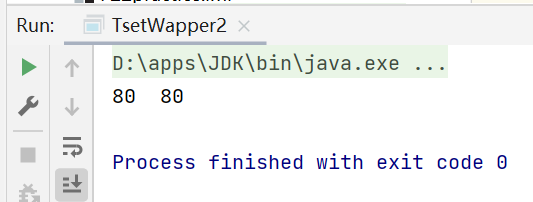1. equals与==的区别：
1. ==是判断两个变量或实例是不是指向同一个内存地址，equals是判断两个变量或实例所指向的内存空间的值是不是相同
2. ==是指对内存地址进行比较 ，equals()是对字符串的内容进行比较
3. ==指引用是否相同， equals()指的是值是否相同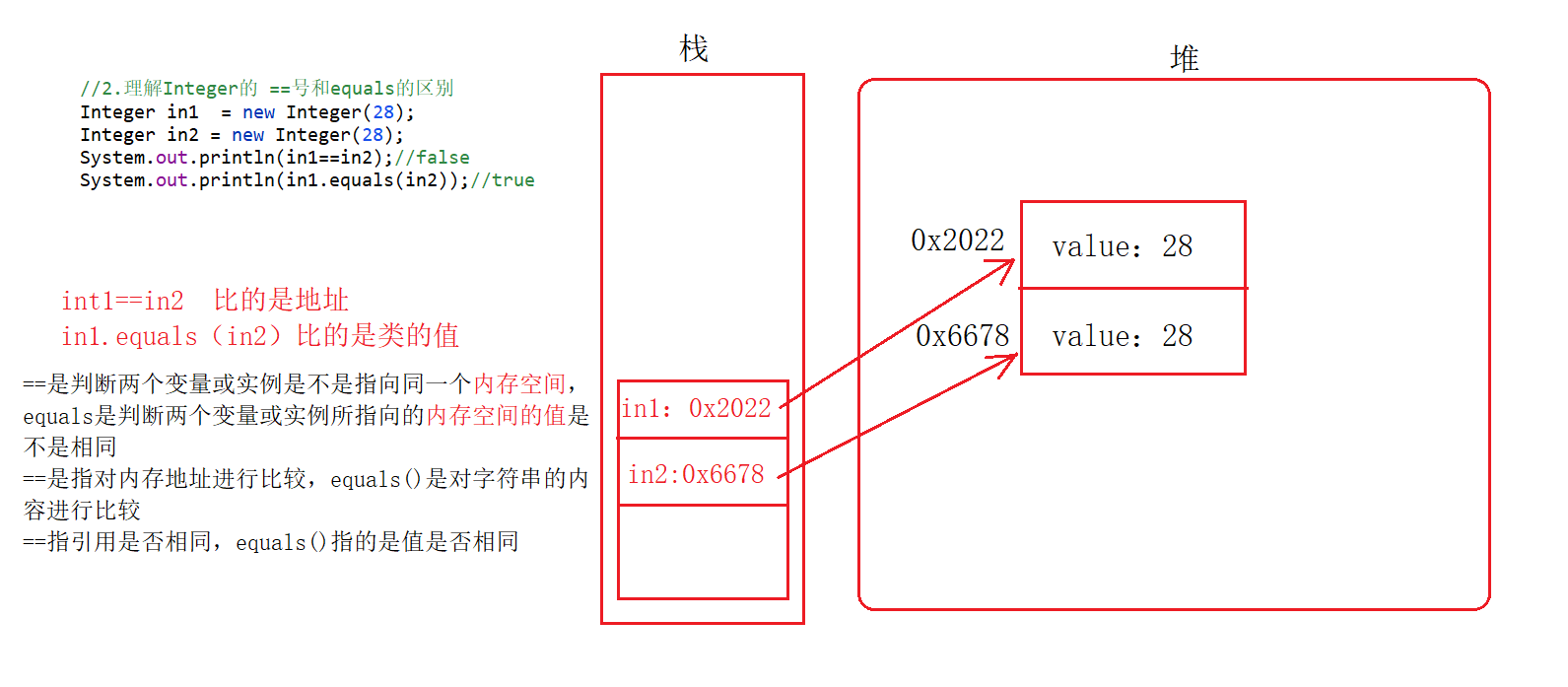1. Integer.valueOf();//自动装箱底层代码源码：
``````public static Integer valueOf(int i) {
if (i >= IntegerCache.low && i <= IntegerCache.high)
return IntegerCache.cache[i + (-IntegerCache.low)];
return new Integer(i);
}
``````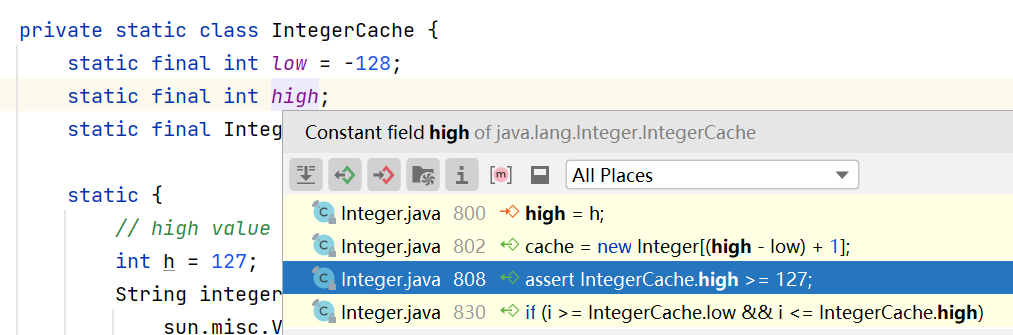Integer类提供了一个静态内部类IntegerCache，对于定义一个静态内部类cache，长度为256，赋值为-128-127。对于自动装箱是如果在此范围内的数据，则直接获取数组的指定值；对于这个范围外的数据，通过new Integer（）重新创建对象。这么做目的是提高效率。

``````package li.normalclass.warpper;

public class TsetWapper2 {
public static void main(String[] args) {
//2.理解Integer的 ==号和equals的区别
Integer in1  = new Integer(280);//手动装箱
Integer in2 = new Integer(280);//手动装箱
System.out.println(in1==in2);//false，引用类型 ==比较的是地址
System.out.println(in1.equals(in2));//true，比较的是对象内存空间中的值中的值

Integer in3 = -127;
Integer in4 = -127;

System.out.println(in1==in2);//true!!!  [-128~127]之间，基本数据类型，比较的是值的大小

System.out.println(in1.equals(in2));//true，比较的是内存空间中的值
/*原因：
Integer in3 = Integer.valueOf(-127);//自动装箱的真实代码
Integer in4 = Integer.valueOf(-127);

Integer类提供了一个静态内部类IntegerCache，对于定义一个静态内部类cache，长度为256，赋值为-128-127。对于自动装箱是如果在此范围内的数据，则直接获取数组的指定值；对于这个范围外的数据，通过new Integer（）重新创建对象。这么做目的是提高效率。
System.out.println(in1==in2);
这里int1和in2指向的都是数组中-127指向的下标地址，因此比较结果为true

*/

Integer in5 = 1234;
Integer in6 = 1234;
System.out.println(in3 == in4);//false，比较的是内存地址
System.out.println(in3.equals(in4));//true，比较的是对象中的值

}
}
``````